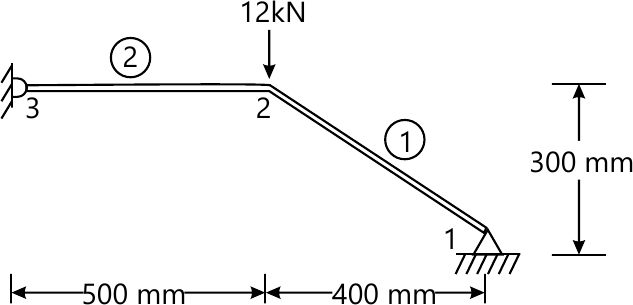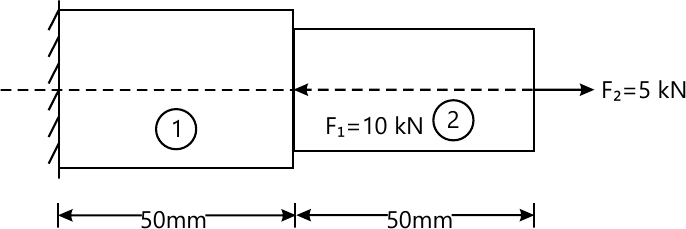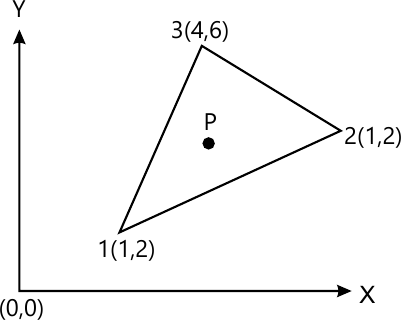MORE IN Finite Element Analysis
MU Mechanical Engineering (Semester 8)
Finite Element Analysis
May 2012
Total marks: --
Total time: --
INSTRUCTIONS
(1) Assume appropriate data and state your reasons
(2) Marks are given to the right of every question
(3) Draw neat diagrams wherever necessary

1(a) Attempt any four of the following:
Briefly explain application of FEM in Various fields.
5 M
1(b) Explain principle of minimum Potential Energy.
5 M
1(c) Explain different sources of error in a typical F.E.M solution.
5 M
1(d) Briefly explain Node Numbering Scheme.
5 M
1(e) Explain properties of Global Matrix.
5 M

2(a) Solve the following differential equation using Galerkin's method $3\dfrac{d^{2}u}{dx^{2}}-3u+4x^{2}=0$ with boundary condition u(o) =u(1) =0.Assume Cubic polynomial for approximate solution.
10 M
2(b) Solve the following differential equation using Rayleigh-Ritz method $3\dfrac{d^{2}y}{dx^{2}}-\dfrac{dy}{dx}+8=0\ \cdots\ 0≤x≤1$ with boundary condition y(0) =1 and y(1) =2.assume cubic Polynomial for trial solution. Find the value at y(2,3) and y(0,8)
10 M

$I=\int_{-1}^{1} \int_{-1}^{1}(r^{3}-1)(s-1)^{2}dr ds$
 n ϵ w 1 0.0 2 2 ± 0.5774 1 3 ± 0.0 ± 0.7746 0.8889 0.5556
12 M
3(b) Explain the following:
Convergence requirements
Global,local and natural co-ordinate system
8 M

4(a) For the bar truss shown in figure, determine the nodal displacement, stresses in each element and reaction at support. Take $E =2\times 10^{5} \dfrac{N}{mm^{2}}, A= 200mm^{2}$15 M
4(b) Explain Band width.
8 M

5(a) Using Direct Stiffness method,determine the nodal displacements of stepped bar shown in12 M
5(b) Derive the shape function for a Quadratic bar element [3 noded 1 dimensional bar]using Lagrangian polynomial in,
Global co-ordinates and
Natural co-ordinates.
8 M

6(a) Find the shape function for two dimensional Nine rectangular elements mapped into natural coordinates.
12 M
6(b) The nodal co-ordinates of a triangular element are as shown in figure.The x co-ordinate of interior point P is 3.3 and shape function N1=0.3.Determine N2 N3 and y co-ordinates of point P8 M

7(a) Find the natural frequency of axial vibration of a bar of uniform cross section of 20mm2 and length 1m. Take $E=2\times 10^{5} \dfrac{N}{mm^{2}}$ and $\rho =8000\dfrac{kg}{m^{3}}$
Take 2 linear elements.
10 M
7(b) Discuss briefly higher order and iso-parametric elements with suitable sketches.
10 M

More question papers from Finite Element Analysis